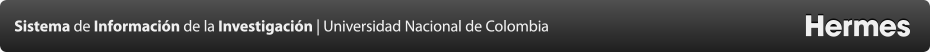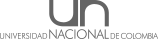Proyectos
Nonlinear forecasting of stream flows using chaotic approach and artificial neural networks
Resumen
This paper evaluates the forecasting performances of two nonlinear models, i.e. k-nearest neighbor (kNN) and feed-forward neural networks (FFNN) in stream flow data of K¿z¿l¿rmak River, the longest river of Turkey. For the kNN model, the required parameters are delay time, number of nearest neighbor number and embedding dimension. The optimal delay time was obtained with the mutual information function and the number of nearest neighbor was obtained with the optimization process that minimizes RMSE as a function of neighbor number. And the embedding dimension was obtained with the correlation dimension method. The correlation dimension of K¿z¿l¿rmak River is obtained as and further it was used in forming of input structure of FFNN. The nearest integer above the correlation dimension (i.e. 3) provides the minimal number of required variables and the maximum number of required variables to characterize the system is obtained with (Takens, 1981) (i.e. 7). Two FFNN models were developed that incorporates 3 and 7 lagged discharge values and the prediction performances compared with the kNN model. The results showed that the kNN model is superior over FFNN in stream flow forecasting. However, resulted from the kNN model structure, the model failed in prediction of peak values. Also, it was found that the correlation dimension can successfully be used (if exists) in time series where the determination of input structure is difficult because of high inter-dependency as in stream flow time series.
Convocatoria
 Nombre de la convocatoria: CONVOCATORIA DEL PROGRAMA NACIONAL PARA LA VISIBILIDAD INTERNACIONAL DE LA PRODUCCIÓN ACADÉMICA MEDIANTE EL APOYO PARA TRADUCCIÓN O CORRECCIÓN DE ESTILO DE ARTÍCULOS DE INVESTIGACIÓN 2013-2015 Modalidad: Modalidad 2. Revisión de estilo de artículos completos, o traducción o revisión de estilo de resúmenes de artículos, que hayan sido aprobados para su publicación e integren un número próximo de revistas de la Universidad Nacional de Colombia indexadas en el IBN-Publindex.
Responsable